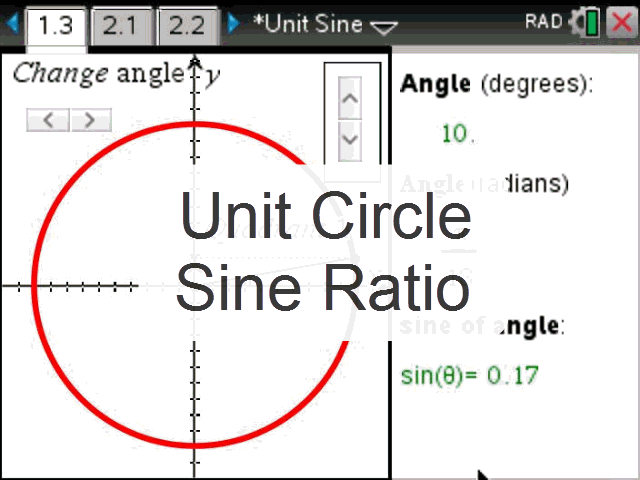# Activities

•• ##### Subject Area

• Mathematics: Function and Graphs: Function and Graphs
• Standard: ACT - Year 12 Certificate: Mathematics Methods: Mathematics Methods
• Standard: QLD - Senior Certificate: Mathematics B: Mathematics B
• Standard: VCE: Mathematical Methods: Mathematical Methods

• ##### AuthorAust Senior

50 Minutes

• ##### Device
• TI-Nspire™ CAS
• TI-Nspire™ Navigator™
• TI-Nspire™ Apps for iPad®
• ##### Software

TI-Nspire™ CAS
TI-Nspire™ CAS Navigator™ NC System

4.0

## Unit Circle Sine#### Activity Overview

Students explore an animated unit circle, looking for patterns. The interactive content allows students to control the level of assistance when answering questions. To understand relationships such as –sin(x)=sin(-x) switch the hint on to see a triangle with its corresponding reflection. The graph of sin(x) is broken into four parts to align with each quadrant of the unit circle and finally, exact angles are reviewed.

#### Objectives

Explore the connection between the unit circle and periodic patterns in the sine ratio and align these with the graph of sin(x).

#### Vocabulary

Unit circle, exact value, sine,

#### About the Lesson

Students start by passively observing an animation of the unit circle with a view to identifying some of the periodic patterns that occur in the sine ratio. These observations turn a literal response into a rule with the assistance of CAS. Patterns from the unit circle are then applied to a basic graph of sin(x) that is broken up into the respective quadrants followed by practicing calculations with exact angles and the material learnt to date.